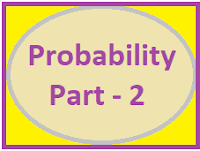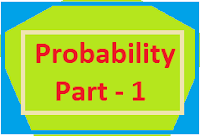## Posts

Showing posts from December, 2018

### Resource Centre Mathematics

Resource Centre Mathematics Mathematics worksheet, mathematics basic points and formulas, mathematics lesson plan, mathematics multiple choice questions Workplace Dashboard CBSE Syllabus For Session 2023-24 For  :   Classes IX & X    |   Classes XI & XII Watch Videos on Maths Solutions CLASS IX MATHEMATICS FORMULAS &  BASIC CONCEPTS

### PROBABILITY Part-2PROBABILITY PART-2 This part is the continuation of Probability part-1. This Post is specially for the students after 10th standard. For complete knowledge first students study Probability Part-1 and then part-2 Main points to be discussed here 1 Random Experiment 2 Different types of events 3 Variable description of the events 4 Conditional Probability 5 Multiplication Theorem on Probability 6 Laws of Total Probability 7 Baye's Theorem 8 Bernoulli's Trial 9 Mean and Variance of the Distribution 10 Shortcut Method of finding Mean, Variance and Standard Deviation. Random Experiment:-   An experiment whose outcomes cannot be predicted or determined in advance are called a random experiment. Elementary events:-   If a random e

### PROBABILITY Part-1PROBABILITY  For classes 8th, 9th, 10th, 11th Basic concepts of probability useful for classes 9, 10 and 11. Explanation with playing cards, tossing one coin, two coins and three coins. Probability of an event        =   If E is an event then :-  P(E) + P(notE) = 1 Probability of an event is greater than, equal to 0 but is less than, equal to 1. This is also be called as the range of Probability $0\leq P(E)\leq 1$ Impossible Event:-   An event which never occur is called impossible event.  P(Impossible Event) = 0 Sure Event : An even which happens for sure is called sure event. Sure event is also be called as the certain event. P(Sure Event or Certain Event) = 1 Equally likely events :-   If every event of the sample space has equal possibility to happen, then the events are called equally likely events. (e.g.  Tossing a coin) PROBABILITY WITH PLAYING CARDS             DESCRIPTION OF PLAYING CARDS